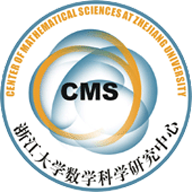(07003) New results of intersection numbers on moduli spaces of curves

Abstract

We present a series of new results we obtained recently about the intersection numbers of tautological classes on moduli spaces of curves, including a simple formula of the n-point functions for Witten's $\tau$ classes, an effective recursion formula to compute higher Weil-Petersson volumes, several new recursion formulae of intersection numbers and our proof of a conjecture of Itzykson and Zuber concerning denominators of intersection numbers. We also present Virasoro and KdV properties of generating functions of general mixed $\kappa$ and $\psi$ intersections.

• IntersectionNumbers.pdf